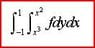# Reversing order of integration of double integral qns.

• blursotong
yup.i got them now. i get (jus for the -ve x portion)-1 < x < -sqrt y with 0< y< 1and -1 < x < -cube root y with -1< y < 0

#### blursotong

[sloved]reversing order of integration of double integral qns.

## Homework Statement

pls refer to attached picture.

## The Attempt at a Solution

intially upper and lower limits are , x^2 < y< x^3 and -1<x<1
sketched y=x^2 and y= x^3. => sqrt(y) =x and cube root (y) = x
divide the area into 3 section.
new limits of dxdy
sqrt(y) <x< cube root (y) with 0<y<1 ,
and 0<x< sqrt(y) with 0<y< -1, ( for -ve x and +ve y portion of x^2 graph)
and cube root (y)< x< 0 with -1<y<0 ( for -ve x and -ve y portion of x^3 graph)

but the answer i have shows a different answer. guess i am wrong, but anyone can tell me which part?
attached is a graph i tried to draw( pardon my IT skills=P)

#### Attachments

•Capture.JPG
2.7 KB · Views: 348
• Doc1.doc
32 KB · Views: 195
Last edited:
Your picture is good, but you should emphasize that the region is also bounded by a vertical segment at x=(-1). Doesn't that mean some of your lower limits in the x integration need to be -1?

hmm..to find limit of x integration we draw vertical line? so when i split the area to three sections, their upper limits is cube root y or sqrt y and the lower limit is 0?
yea, but according to the answer i have, it uses limits of x integration as -1..and i don't understand why?
could you kindly explain to me?

Take your diagram and draw a segment connecting (-1,1) and (-1,-1). That's the boundary curve that determines your lower limit when you are doing the x<0 parts of the integration.

opps..i was wrong, we should draw horizontal lines instead. and yup..
i get jus (for the -ve x portion)
-1 < x < sqrt y with 0< y< 1
and -1 < x < -x^3 with -1< y < 0
using the these lines as a guide..

opps i only can attach files when i post a thread? can't seem to be able to upload the edited graph here??

blursotong said:
opps..i was wrong, we should draw horizontal lines instead. and yup..
i get jus (for the -ve x portion)
-1 < x < sqrt y with 0< y< 1
and -1 < x < -x^3 with -1< y < 0
using the these lines as a guide..

You'll need to express x limits in terms of y. And for both of them, the upper limit should be a negative number, like your graph shows.

yup.i got them now.
i get (jus for the -ve x portion)
-1 < x < -sqrt y with 0< y< 1
and -1 < x < -cube root y with -1< y < 0

thanks a lot for your guidance=D

blursotong said:
yup.i got them now.
i get (jus for the -ve x portion)
-1 < x < -sqrt y with 0< y< 1
and -1 < x < -cube root y with -1< y < 0

thanks a lot for your guidance=D

Are you super sure about that -cube root(y)?? Remember if y is negative then cube root(y) is already negative.

ahah! my wrong..it should be jus cube root of y...
thx for pointing out!
=D